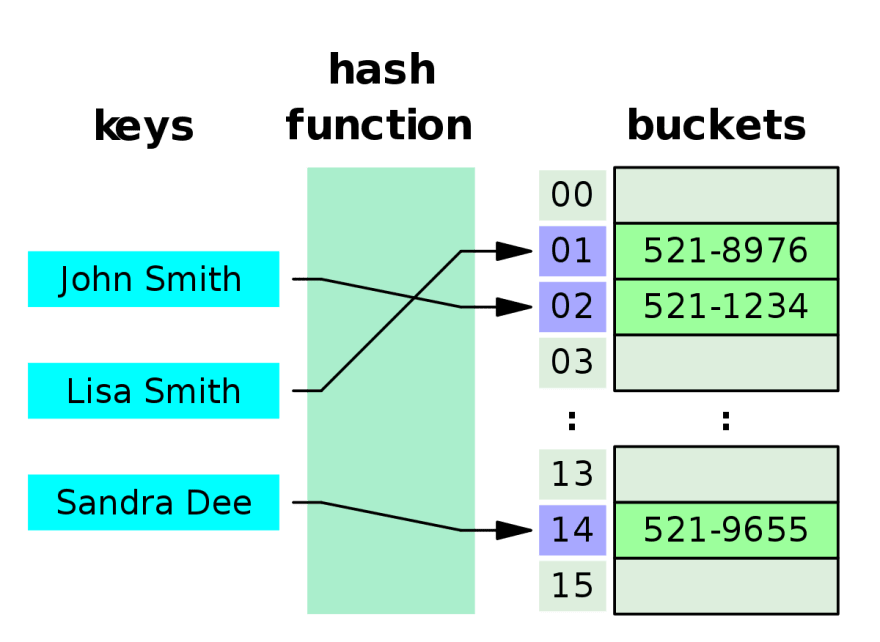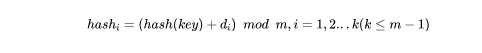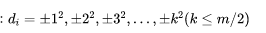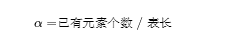### Python数据结构：哈希表• 若关键字为 ，则其值存放在的存储位置上。由此，不需比较便可直接取得所查记录。称这个对应关系  为散列函数，按这个思想建立的表为散列表。

• 对不同的关键字可能得到同一散列地址，即 ，而 ，这种现象称为冲突。

• 若对于关键字集合中的任一个关键字，经散列函数映象到地址集合中任何一个地址的概率是相等的，则称此类散列函数为均匀散列函数（Uniform Hash function），这就是使关键字经过散列函数得到一个“随机的地址”，从而减少冲突。• 线性探测法：，或者其他线性函数。相当于逐个探测存放地址的表，直到查找到一个空单元，然后放置在该单元。

• 平方探测法：• 查字典

• 网络防火墙中，根据源IP，目的IP，源端口，目的端口，协议号构成的五元组来标识一条网络数据流的，并且根据五元组来建立会话表项(session entry)。为了查找便捷，一般都使用Hash表来实现这个会话表，以提高转发的效率。

• Linux 内核大量采用哈希表

``class Hash:    # 表的长度定位11    def __init__(self):        self.hash_table=[[None,None]for i in range(11)]        # 散列函数    def hash(self,k,i):        h_value=(k+i)%11        if self.hash_table[h_value]==k:            return h_value        if self.hash_table[h_value]!=None:            i+=1            h_value=self.hash(k,i)        return h_value        def put(self,k,v):        hash_v=self.hash(k,0)        self.hash_table[hash_v]=k        self.hash_table[hash_v]=v     def get(self,k):        hash_v=self.hash(k,0)        return self.hash_table[hash_v] hash = Hash()hash.put(1 ,'wang')print(hash.get(1))````class Map:    def __init__(self):        self.capacity=11        self.hash_table=[[None,None]for i in range(self.capacity)]        self.num=0        self.load_factor=0.75        def hash(self,k,i):        h_value=(k+i)%self.capacity        if self.hash_table[h_value]==k:            return h_value        if self.hash_table[h_value]!=None:            i+=1            h_value=self.hash(k,i)        return h_value     def resize(self):         #扩容到原有元素数量的两倍        self.capacity=self.num*2        temp=self.hash_table[:]        self.hash_table=[[None,None]for i in range(self.capacity)]         for i in temp:             #把原来已有的元素存入            if(i!=None):                hash_v=self.hash(i,0)                self.hash_table[hash_v]=i                self.hash_table[hash_v]=i     def put(self,k,v):        hash_v=self.hash(k,0)        self.hash_table[hash_v]=k        self.hash_table[hash_v]=v        #暂不考虑key重复的情况，具体自己可以优化        self.num+=1        # 如果比例大于载荷因子        if(self.num/len(self.hash_table)>self.load_factor):            self.resize()     def get(self,k):        hash_v=self.hash(k,0)        return self.hash_table[hash_v]``

Python 中的字典就是用哈希表来实现的，它的特点如下：

• 字典的每个键值 key=>value 对用冒号 : 分割，每个键值对之间用逗号 , 分割，整个字典包括在花括号 {} 中 ,格式：dict = {key1 : value1, key2 : value2 }

• 通过中括号访问，添加，更新元素

``dictionary = {'name': 'wang', 'age': 17, 'class': 'first'}dictionary['age'] = 18dictionary['country'] = 'china'print(dictionary['age'])print(dictionary['country'])``

``class MyDictionary(object):    # 字典类的初始化    def __init__(self):        self.table_size = 13 # 哈希表的大小        self.key_list = [None]*self.table_size #用以存储key的列表        self.value_list = [None]*self.table_size #用以存储value的列表        # 散列函数，返回散列值    # key为需要计算的key    def hashfuction(self, key):        count_char = 0        key_string = str(key)        for key_char in key_string: # 计算key所有字符的ASCII值的和            count_char += ord(key_char) # ord()函数用于求ASCII值        length = len(str(count_char))        if length > 3 : # 当和的位数大于3时，使用平方取中法，保留中间3位            mid_int = 100*int((str(count_char)[length//2-1])) \                    + 10*int((str(count_char)[length//2])) \                    + 1*int((str(count_char)[length//2+1]))        else: # 当和的位数小于等于3时，全部保留            mid_int = count_char                    return mid_int%self.table_size # 取余数作为散列值返回            # 重新散列函数，返回新的散列值    # hash_value为旧的散列值    def rehash(self, hash_value):        return (hash_value+3)%self.table_size #向前间隔为3的线性探测            # 存放键值对    def __setitem__(self, key, value):        hash_value = self.hashfuction(key) #计算哈希值        if None == self.key_list[hash_value]: #哈希值处为空位，则可以放置键值对            pass        elif key == self.key_list[hash_value]: #哈希值处不为空，旧键值对与新键值对的key值相同，则作为更新，可以放置键值对            pass        else: #哈希值处不为空，key值也不同，即发生了“冲突”，则利用重新散列函数继续探测，直到找到空位            hash_value = self.rehash(hash_value) # 重新散列            while (None != self.key_list[hash_value]) and (key != self.key_list[hash_value]): #依然不能插入键值对，重新散列                hash_value = self.rehash(hash_value) # 重新散列        #放置键值对              self.key_list[hash_value] = key        self.value_list[hash_value] = value     # 根据key取得value    def __getitem__(self, key):        hash_value = self.hashfuction(key) #计算哈希值        first_hash = hash_value #记录最初的哈希值，作为重新散列探测的停止条件        if None == self.key_list[hash_value]: #哈希值处为空位，则不存在该键值对            return None        elif key == self.key_list[hash_value]: #哈希值处不为空，key值与寻找中的key值相同，则返回相应的value值            return self.value_list[hash_value]        else: #哈希值处不为空，key值也不同，即发生了“冲突”，则利用重新散列函数继续探测，直到找到空位或相同的key值            hash_value = self.rehash(hash_value) # 重新散列            while (None != self.key_list[hash_value]) and (key != self.key_list[hash_value]): #依然没有找到，重新散列                hash_value = self.rehash(hash_value) # 重新散列                if hash_value == first_hash: #哈希值探测重回起点，判断为无法找到了                    return None            #结束了while循环，意味着找到了空位或相同的key值            if None == self.key_list[hash_value]: #哈希值处为空位，则不存在该键值对                return None            else: #哈希值处不为空，key值与寻找中的key值相同，则返回相应的value值                return self.value_list[hash_value]        # 删除键值对    def __delitem__(self, key):        hash_value = self.hashfuction(key) #计算哈希值        first_hash = hash_value #记录最初的哈希值，作为重新散列探测的停止条件        if None == self.key_list[hash_value]: #哈希值处为空位，则不存在该键值对，无需删除            return        elif key == self.key_list[hash_value]: #哈希值处不为空，key值与寻找中的key值相同，则删除            self.key_list[hash_value] = None            self.value_list[hash_value] = None            return        else: #哈希值处不为空，key值也不同，即发生了“冲突”，则利用重新散列函数继续探测，直到找到空位或相同的key值            hash_value = self.rehash(hash_value) # 重新散列            while (None != self.key_list[hash_value]) and (key != self.key_list[hash_value]): #依然没有找到，重新散列                hash_value = self.rehash(hash_value) # 重新散列                if hash_value == first_hash: #哈希值探测重回起点，判断为无法找到了                    return            #结束了while循环，意味着找到了空位或相同的key值            if None == self.key_list[hash_value]: #哈希值处为空位，则不存在该键值对                return            else: #哈希值处不为空，key值与寻找中的key值相同，则删除                self.key_list[hash_value] = None                self.value_list[hash_value] = None                return        # 返回字典的长度    def __len__(self):        count = 0        for key in self.key_list:            if key != None:                count += 1        return count def main():    H = MyDictionary()    H["kcat"]="cat"    H["kdog"]="dog"    H["klion"]="lion"    H["ktiger"]="tiger"    H["kbird"]="bird"    H["kcow"]="cow"    H["kgoat"]="goat"    H["pig"]="pig"    H["chicken"]="chicken"    print("字典的长度为%d"%len(H))    print("键 %s 的值为为 %s"%("kcow",H["kcow"]))    print("字典的长度为%d"%len(H))    print("键 %s 的值为为 %s"%("kmonkey",H["kmonkey"]))    print("字典的长度为%d"%len(H))    del H["klion"]    print("字典的长度为%d"%len(H))    print(H.key_list)    print(H.value_list)    if __name__ == "__main__":    main()``